## Species-specific audio detection: a comparison of three template-based detection algorithms using random forests

Note that a Preprint of this article also exists, first published January 11, 2017.

## Introduction

Monitoring fauna is an important task for ecologists, natural resource managers, and conservationists. Historically, most data were collected manually by scientists that went to the field and annotated their observations (Terborgh et al., 1990). This generally limited the spatial and temporal extend of the data. Furthermore, given that the data were based on an individual’s observations, the information was difficult to verify, reducing its utility for understanding long-term ecological processes (Acevedo & Villanueva-Rivera, 2006).

To understand the impacts of climate change and deforestation on the fauna, the scientific community needs long-term, wide-spread and frequent data (Walther et al., 2002). Passive acoustic monitoring (PAM) can contribute to this need because it facilitates the collection of large amounts of data from many sites simultaneously, and with virtually no impact to the fauna and environment (Brandes, 2008; Lammers et al., 2008; Tricas & Boyle, 2009; Celis-Murillo, Deppe & Ward, 2012). In general, PAM systems include a microphone or a hydrophone connected to a self powered system and enough memory to store various weeks or months of recordings, but there are also permanent systems that use solar panels and an Internet connection to upload recordings in real time to a cloud based analytical platform (Aide et al., 2013).

Passive recorders can easily create a very large data set (e.g., 100,000s of recordings) that is overwhelming to manage and analyze. Although researchers often collect recordings twenty-four hours a day for weeks or months (Acevedo & Villanueva-Rivera, 2006; Brandes, 2008; Lammers et al., 2008; Sueur et al., 2008; Marques et al., 2013; Blumstein et al., 2011), in practice, most studies have only analyzed a small percentage of the total number of recordings.

Web-based applications have been developed to facilitate data management of these increasingly large datasets (Aide et al., 2013; Villanueva-Rivera & Pijanowski, 2012), but the biggest challenge is to develop efficient and accurate algorithms for detecting the presence or absence of a species in many recordings. Algorithms for species identification have been developed using spectrogram matched filtering (Clark, Marler & Beeman, 1987; Chabot, 1988), statistical feature extraction (Taylor, 1995; Grigg et al., 1996), k-Nearest neighbor algorithm (Han, Muniandy & Dayou, 2011; Gunasekaran & Revathy, 2010), Support Vector Machine (Fagerlund, 2007; Acevedo et al., 2009), tree-based classifiers (Adams, Law & Gibson, 2010; Henderson, Hildebrand & Smith 2011) and template based detection (Anderson, Dave & Margoliash, 1996; Mellinger & Clark, 2000), but most of these algorithms are built for a specific species and there was no infrastructure provided for the user to create models for other species.

In this study, we developed a method that detects the presence or absence of a species’ specific call type in recordings with a response time that allows researchers to create, run, tune and re-run models in real time as well as detect hundreds of thousands of recordings in a reasonable time. The main objective of the study was to compare the performance (e.g., efficiency and accuracy) of three variants of a template-based detection algorithm and incorporate the best into the ARBIMON II bioacoustics platform. The first variant is the Structural Similarity Index described in Wang et al. (2004), a widely use method to find how similar two images are (in our case the template with the tested recording). The second method filters the recordings with the dynamic thresholding method described in Van der Walt et al. (2014) and then use the Frobenius norm to find similarities with the template. The final method uses the Structural Similarity Index, but it is only applied to regions with high match probability determined by the OpenCV’s matchTemplate procedure (Bradski, 2000).

## Materials and Methods

### Passive acoustic data acquisition

We gathered recordings from five locations: four in Puerto Rico and one in Peru. Some of the recordings were acquired using the Automated Remote Biodiversity Monitoring Network (ARBIMON) data acquisition system described in Aide et al. (2013), while others were acquired using the newest version of ARBIMON permanent recording station, which uses an Android cell phone and transmits the recorded data through a cellular network. All recordings have a sampling rate of 44.1 kHz, a sampling depth of 16-bit and an approximate duration of 60 s (±.5 s)

The locations in Puerto Rico were the Sabana Seca permanent station in Toa Baja, the Casa la Selva station in Carite Mountains (Patillas), El Yunque National Forest in Rio Grande and Mona Island (see Fig. 1). The location in Peru was the Amarakaeri Communal Reserve in the Madre de Dios Region (see Fig. 2). In all the locations, the recorders were programmed to record one minute of audio every 10 min. The complete dataset has more than 100,000 1-minute recordings. We randomly chose 362 recordings from Puerto Rico and 547 recordings from Peru for comparing the three algorithm variants.

We used the ARBIMON II web application interface to annotate the presence or absence of 21 species in all the recordings. Regions in the recording where a species emits a sound were also marked using the web interface. Each region of interest (ROI) is a rectangle delimited by starting time, ending time, lowest frequency and highest frequency along with a species and sound type. The species included in the analysis are listed in Table 1, along with the number of total recordings and the number of recordings where the species is present or absent.

### Algorithm

The algorithm recognition process is divided into three phases: (1) Template Computation, (2) Model Training and (3) Detection (see Fig. 3). In Template computation, all ROIs submitted by the user in the training set are aggregated into a template. In Model Training the template is used to compute recognition functions from validated audio recordings and features from the resulting vector V are computed. These features are used to train a random forest model. In the Detection phase the template is used to compute the features, but this time the features are fed to the trained random forest model to compute a prediction of presence or absence.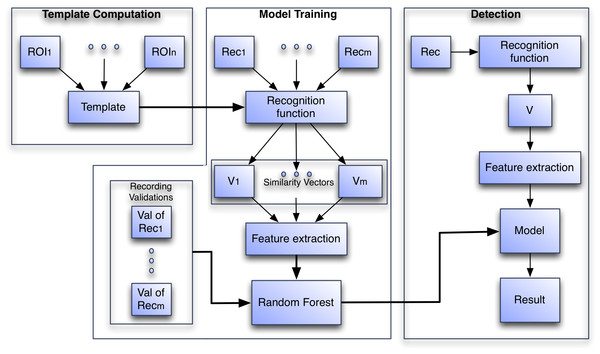Figure 3: The three phases of the algorithm to create the species-specific models. In the Model Training phase Reci is a recording, Vi is the vector generated by the recognition function on Reci and in the Detection phase V is the vector generated by the recognition function on the incoming recording.   Download full-size image DOI: 10.7717/peerjcs.113/fig-3

In the following sections the Template Computation process will be explained, then the process of using the Template to extract features from a recording is presented and finally, the procedures to use the features to train the model and to detect recordings are discussed.

#### Template computation

The template refers to the combination of all ROIs in the training data. To create a template, we first start with the examples of the specific call of interest (i.e., ROIs) that were annotated from a set of recordings for a given species and a specific call type (e.g., common, alarm). Each ROI encompasses an example of the call, and is an instance of time between time t1 and time t2 of a given recording and low and high boundary frequencies of f1 and f2, where t1 < t2 and f1 < f2. In a general sense, we combine these examples to produce a template of a specific song type of a single species.

Specifically, for each recording that has an annotated ROI, a spectrogram matrix (SM) is computed using the Short Time Fourier Transform with a frame size of 1024 samples, 512 samples of overlap and a Hann analysis window, thus the matrices have 512 rows. For a recording with a sampling rate of 44,100 Hz, the matrix bin bandwidth is approximately 43.06 Hz. The SM is arranged so that the row of index 0 represents the lowest frequency and the row with index 511 represents the highest frequency of the spectrum. Properly stated the columns c1 to c2 and the rows from r1 to r2 of SM were extracted, where: ${c}_{1}=⌊{t}_{1}×44,100⌋,\phantom{\rule{20.00003pt}{0ex}}{c}_{2}=⌊{t}_{2}×44,100⌋,\phantom{\rule{20.00003pt}{0ex}}{r}_{1}=⌊{f}_{1}∕43.06⌋\phantom{\rule{10.00002pt}{0ex}}\text{and}\phantom{\rule{10.00002pt}{0ex}}{r}_{2}=⌊{f}_{2}∕43.06⌋.$ The rows and columns that represent the ROI in the recording (between frequencies f1 and f2 and between times t1 and t2) are extracted. The submatrix of SM that contains only the area bounded by the ROI is define as SMROI and refer in the manuscript as the ROI matrix.

Since the ROI matrices can vary in size, to compute the aggregation from the ROI matrices we have to take into account the difference in the number of rows and columns of the matrices. All recordings have the same sampling rate, 44,100 Hz. Thus, the rows from different SMs, computed with the same parameters, will represent the same frequencies, i.e., rows with same indexes represent the same frequency. After the ROI matrix, SMROI, has been extracted from SM, the rows of SMROI will also represent specific frequencies. Thus, if we were to perform an element-wise matrix sum between two ROI matrices with potentially different number of rows, we should only sum rows that represent the same frequency.

To take into account the difference in the number of columns of the ROI matrices, we use the Frobenius norm to optimize the alignment of the smaller ROI matrices and perform element-wise sums between rows that represent the same frequency. We present that algorithm in the following section and a flow chart of the process in Fig. 4.

#### Template computation algorithm:

1. Generate the set of SMROI matrices by computing the short time Fourier Transform of all the user generated ROIs.

2. Create matrix SMmax, a duplicate of the first created matrix among the matrices with the largest number of columns.

3. Set cmax as the number of columns in SMmax.

4. Create matrix Ttemp, with the same dimensions as SMmax and all entries equal to 0. This matrix will contain the element-wise addition of all the extracted SMROI matrices.

5. Create matrix W with the same dimensions of SMmax and all entries equal to 0. This matrix will hold the count on the number of SMROI matrices that participate in the calculation of each element of Ttemp.

6. For each one of the SMi ROI matrices in SMROI:

1. If SMi has the same number of columns as Ttemp:

1. Align the rows of SMi and Ttemp so they represent equivalent frequencies and perform an element-wise addition of the matrices and put the result in Ttemp.

2. Add one to all the elements of the W matrix where the previous addition participated.

2. If the number of columns differs between SMi and Ttemp, then find the optimal alignment with SMmax as follows:

1. Set ci as the number of columns in SMi.

2. Define (SMmax)I as the set of all submatrices of SMmax with the same dimensions as SMi. Note that the cardinality of (SMmax)I is cmax − ci.

3. For each Subk ∈ (SMmax)I:

1. Compute dk = NORM(Subk − SMi) where NORM is the Frobenius norm defined as: $NORM\left(A\right)=\sqrt{\sum _{\left(i,j\right)}|{a}_{i,j}^{2}|}$ where ai,j are the elements of matrix A.

4. Define Submin{dk} as the Subk matrix with the minimum dk. This is the optimal alignment of SMi with SMmax.

5. Align the rows of Submin{dk} and Ttemp so they represent equivalent frequencies, perform an element-wise addition of the matrices and put the result in Ttemp.

6. Add one to all the elements of the W matrix where the previous addition participated.

7. Define the matrix Ttemplate as the element-wise division between the Ttemp matrix and the W matrix.

The resulting Ttemplate matrix summarizes the information available in the ROI matrices submitted by the user and it will be used to extract information from the audio recordings that are to be analyzed. In this article each species Ttemplate was created using five ROIs.

In Fig. 5A a training set for the Eleutherodactylus coqui is presented and in Fig. 5B the resulting template can be seen. This tool is very useful because the user can see immediately the effect of adding or subtracting a specific sample to the training set.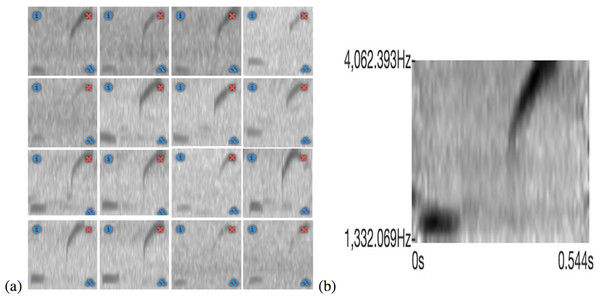Figure 5: (A) A training set with 16 examples of the call of E. coqui. (B) The resulting template from the training set.   Download full-size image DOI: 10.7717/peerjcs.113/fig-5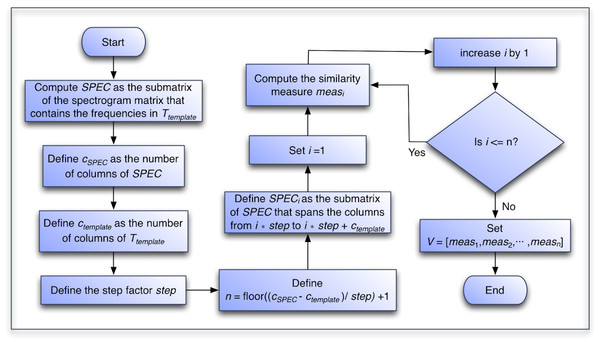Figure 6: Flowchart of the algorithm to generate the similarity vector of each recording.   Download full-size image DOI: 10.7717/peerjcs.113/fig-6

### Model training

The goal of this phase is to train a random forest model. The input to train the random forest are a series of statistical features extracted from vectors Vi that are created by computing a recognition function (similarity measure) between the computed Ttemplate and submatrices of the spectrogram matrices of a series of recordings.

In the following section, we present the details of the algorithm that processes a recording to create the recognition function vector, and in Fig. 6 we present a flowchart of the process.

#### Algorithm to Create the Similarity Vector:

1. Compute matrix SPEC, the submatrix of the spectrogram matrix that contains the frequencies in Ttemplate. Note that we are dealing with recordings that have the same sample rate as the recordings used to compute the Ttemplate.

2. Define cSPEC, the number of columns of SPEC.

3. Define ctemplate, the number of columns of Ttemplate. Note that cSPEC ≫ ctemplate since the SPEC matrix have the same number of columns as the whole spectrogram and that the Ttemplate matrix fits c = cSPEC − ctemplate + 1 times inside the SPEC matrix. There are c submatrices of SPEC with the same dimensions as Ttemplate.

4. Define step, the step factor by which Ttemplate will progressed over the SPEC matrix.

5. Define $n=⌊\frac{{c}_{SPEC}-{c}_{template}}{step}⌋+1$. Note that if step = 1 then n = c. In this work, however, this parameter was selected as step = 16 as a trade-off for speed.1

6. Define SPECi as the submatrix of SPEC that spans the columns from i × step to i × step + ctemplate.

7. Set i = 1.

8. While i ≤ n

1. Compute the similarity measure measi for SPECi (the definition of measi for each of the three variants is provided in the following section).

2. Increase i by 1. Note that this is equivalent to progressing step columns in the SPEC matrix.

9. Define the vector V as the vector containing the n similarity measures resulting from the previous steps. That is, V = [meas1meas2meas3, …, measn].

#### Recognition function

We used three variations of a pattern match procedure to define the similarity measure vector V. First, the Structural Similarity Index described in Wang et al. (2004) and implemented in Van der Walt et al. (2014) as compare_ssim with the default window size of seven unless the generated pattern is smaller. It will be referred in the rest of the manuscript as the SSIM variant. For the SSIM variant we define measi as: $mea{s}_{i}=SSI\left({T}_{template},SPE{C}_{i}\right),$ where SPECi is the submatrix of SPEC that spans the columns from i × step to i × step + ctemplate and the same number of rows as Ttemplate and V = [meas1meas2meas3, …, measn] with $n=⌊\frac{{c}_{SPEC}-{c}_{template}}{step}⌋+1.$

Second, the dynamic thresholding method (threshold_adaptive) described in Van der Walt et al. (2014) with a block size of 127 and an arithmetic mean filter is used over both Ttemplate and SPECi before multiplying them and applying the Frobenius norm and normalized by the norm of a matrix with same dimensions as Ttemplate and all elements equal to one. Therefore, measi for the NORM variant is defined as: $mea{s}_{i}=FN\left(DTM\left({T}_{template}\right)\phantom{\rule{1em}{0ex}}\phantom{\rule{1em}{0ex}}.\ast \phantom{\rule{1em}{0ex}}\phantom{\rule{1em}{0ex}}DTM\left(SPE{C}_{i}\right)\right)∕FN\left(U\right),$ where again SPECi is the submatrix of SPEC that spans the columns from i × step to i × step + ctemplate, FN is the Frobenius norm, DTM is the dynamic thresholding method, U is a matrix with same dimensions as Ttemplate with all elements equal to one and .∗ performs an element-wise multiplication of the matrices. Again, V = [meas1meas2meas3, …, measn] with $n=⌊\frac{{c}_{SPEC}-{c}_{template}}{step}⌋+1.$

Finally, for the CORR variation we first apply the OpenCV’s matchTemplate procedure (Bradski, 2000) with the Normalized Correlation Coefficient option to SPECi, the submatrix of SPEC that spans the columns from i × step to i × step + ctemplate. However, for this variant, SPECi includes two additions rows above and below, thus it is slightly larger than the Ttemplate. With these we can define: $mea{s}_{j,i}=CORR\left({T}_{template},SPE{C}_{j,i}\right)$ where SPECj,i is the submatrix of SPECi that starts at row j (note that there are five such SPECj,i matrices).

Now, we select five points at random from all the points above the 98.5 percentile of measj,i and apply the Structural Similarity Index to the 5 strongly-matching regions. The size of these regions is eight thirds (8∕3) of the length of Ttemplate, 4∕3 before and 4∕3 after the strongly-matched point that was selected. Then, define FilterSPEC as the matrix that contains these 5 strongly-matching regions and FilterSPECi as the submatrix of FilterSPEC that spans the columns from i to i + ctemplate then, the similarity measure for this variant is define as: $mea{s}_{i}=SSI\left({T}_{template},FilterSPE{C}_{i}\right)$ and the resulting vector V = [meas1meas2meas3, …, measn] but this time with $n=5×\left(⌊8∕3×{c}_{template}⌋+1\right).$

It is important to note that no matter which variant is used to calculate the similarity measures, the result will always be a vector of measurements V. The idea is that the statistical properties of these computed recognition functions have enough information to distinguish between a recording that has the target species present and a recording that does not have the target species present. However, notice that since cSPEC, the length of SPEC, is much larger that ctemplate the length of the vector V for the CORR variant is much smaller than the other two.

#### Random forest model creation

After calculating V for many recordings we can train a random forest model. First, we need a set of validated recordings with the specific species vocalization present in some recordings and absent in others. Then for each recording we compute a vector Vi as described in the previous section and extract the statistical features presented in Table 2. These statistical features represent the dataset used to train the random forest model, which will be used to detect recordings for presence or absence of a species call event. These 12 features along with the species presence information are used as input to a random forest classifier with a 1,000 trees.

### Recording detection

Now that we have a trained model to detect a recording, we have to compute the statistical features from the similarity vector V of the selected recording. This is performed in the same way as it was described in the previous section. These features are then used as the input dataset to the previously trained random forest classifier and a label indicating presence or absence of the species in the recording is given as output.

### The experiment

In order to decide which of the three variants should be incorporated into the ARBIMON web-based system, we performed the algorithm explained in the previous section with each of the similarity measures. We computed 10-fold validations on each of the variants to obtained measurements of the performance of the algorithm. In each validation, 90% of the data is used as training and 10% of the data is used as validation data. Each algorithm variant used the same 10-fold validation partition for each species. The measures calculated were the area under the receiver operating characteristic (ROC) curve (AUC), accuracy or correct detection rate (Ac), negative predictive value (Npv), precision or positive predictive value (Pr), sensitivity, recall or true positive rate (Se) and specificity or true negative rate (Sp). To calculate the AUC, the ROC curve is created by plotting the false positive rate (which can be calculated as 1 − specificity) against the true positive rate (sensitivity), then, the AUC is created by calculating the area under that curve. Notice that the further the AUC is from 0.5 the better. The rest of the measures are defined as follows: $Ac=\frac{{t}_{p}+{t}_{n}}{{t}_{p}+{t}_{n}+{f}_{p}+{f}_{n}}\text{,}\phantom{\rule{20.00003pt}{0ex}}Npv=\frac{{t}_{n}}{{t}_{n}+{f}_{n}}\text{,}\phantom{\rule{20.00003pt}{0ex}}Pr=\frac{{t}_{p}}{{t}_{p}+{f}_{p}}\text{,}\phantom{\rule{20.00003pt}{0ex}}Se=\frac{{t}_{p}}{{t}_{p}+{f}_{n}}\phantom{\rule{20.00003pt}{0ex}}\text{and}\phantom{\rule{20.00003pt}{0ex}}Sp=\frac{{t}_{n}}{{t}_{n}+{f}_{p}}$ with tp the number of true positives (number of times both the expert and the algorithm agree that the species is present), tn the number of true negatives (number of times both the expert and the algorithm agree that the species is not present), fp the number of false positives (number of times the algorithm states that the species is present while the expert states is absent) and fn the number of false negatives (number of times the algorithm states that the species is not present while the expert states it is present). Note that accuracy is a weighted average of the sensitivity and the specificity.

Although we present and discuss all measures, we gave accuracy and the AUC more importance because they include information on the true positive and true negative rates. Specifically, AUC is important when the number of positives is different than the number of negatives as is the case with some of the species.

The experiment was performed in a computer with an Intel i7 4790K 4 cores processor at 4.00 GHz with 32 GB of RAM and running Ubuntu Linux. The execution time needed to detect each recording was registered and the mean and standard deviation of the execution times were calculated for each variant of the algorithm. We also computed the quantity of pixels on all the Ttemplate matrices and correlated with the execution time of each of the variants.

A global one-way analysis of variance (ANOVA) was performed on the five calculated measures across all of the 10-fold validations to identify if there was a significant difference between the variants of the algorithm. Then, a post-hoc Tukey HSD comparison test was performed to identify which one of the variants was significantly different at the 95% confidence level. Additionally, an ANOVA was performed locally between the 10-fold validation of each species and on the mean execution time for each species across the algorithm variants to identify if there was any significant execution time difference at the 95% confidence level. Similarly, a post-hoc Tukey HSD comparison test was performed on the execution times.

## Results

The six measurements (area under the ROC curve—AUC, accuracy, negative predictive value, precision, sensitivity and specificity) computed to compared the model across the three variants varied greatly among the 21 species. The lowest scores were among bird species while most of the highest scores came from amphibian species. Table 3 presents a summary of the results of the measurements comparing the three variants of the algorithm (for a detail presentation, see Appendix 1). The NORM variant did not have the highest value for any of the measures summarized in Table 3, while the CORR variant had a greater number of species with 80% or greater for all the measures and an overall median accuracy of 81%. We considered these two facts fundamental for a general-purpose species detection system.

The local species ANOVA suggested that there are significant accuracy differences at the 95% significance level for six of the 21 species studied as well as four in terms of precision and three in terms of specificity (see https://doi.org/10.6084/m9.figshare.4488149.v1). The algorithm variant CORR had a higher mean and median AUC at 78% and 81% respectively, but the SSIM variant seems to be more stable with a standard deviation of 20%. In terms of accuracy, both the SSIM and CORR have higher mean accuracy than the NORM variant. Nevertheless, variant CORR had the highest median accuracy of 81%, which is slightly higher than the median accuracy of the SSIM variant at 76%. In addition, variant CORR had more species with an accuracy of 80% or greater.

In terms of median precision, the three variants had similar values, although in terms of mean precision variants SSIM and CORR have greater values than the NORM variant. Moreover, the median and mean precision of the SSIM variant were only 1% higher than the median and mean precision of the CORR variant. In terms of sensitivity, variants SSIM and CORR had greater values than the NORM variant. It is only in terms of specificity that the CORR variant has greater values than all other variants. Figures 7 and 8 present a summary of these results with whisker graphs.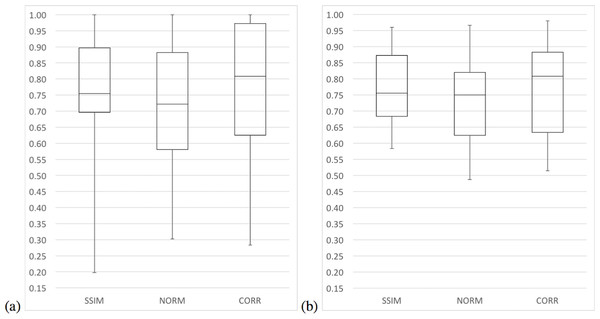Figure 7: Whisker boxes of the 10-fold validations for the three variants of the presented algorithm for: (A) Area under the ROC curve and (B) Accuracy.   Download full-size image DOI: 10.7717/peerjcs.113/fig-7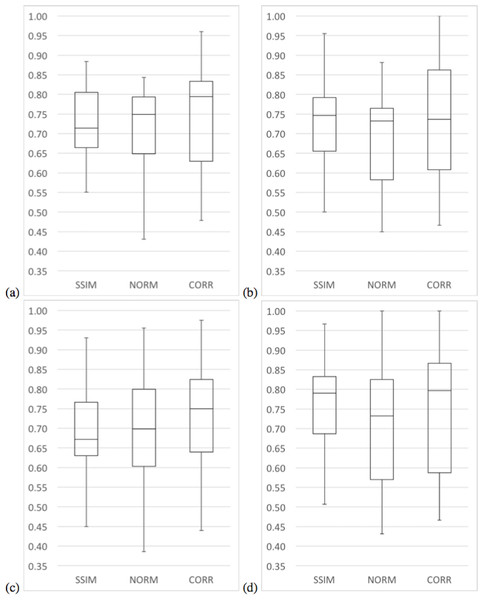Figure 8: Whisker boxes of the 10-fold validations for the three variants of the presented algorithm for: (A) Negative predictive value, (B) Precision, (C) Sensitivity and (D) Specificity.   Download full-size image DOI: 10.7717/peerjcs.113/fig-8

In terms of execution times, an ANOVA analysis on the mean execution time suggests a difference between the variants (F = 9.9341e + 30, df = 3, p < 2.2e–16). The CORR variant had the lowest mean execution time at 0.255 s followed closely by the NORM variant with 0.271 s, while the SSIM variant had the slowest mean execution time of 2.269 s (Fig. 9). The Tukey HSD test suggests that there was no statistical significant difference between the mean execution times of the NORM and CORR variants (p = 0.999). However, there was a statistical significant difference at the 95% confidence level between the mean execution times of all other pairs of variants, specifically variants SSIM and CORR (p < .2e–16).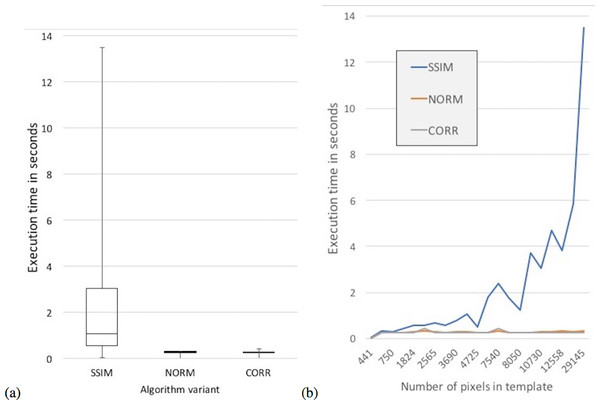Figure 9: (A) Whisker boxes of the execution times of the three algorithms. (B) Execution times as a function of the size of the template in number of pixels.   Download full-size image DOI: 10.7717/peerjcs.113/fig-9

Moreover, the mean execution time of the SSIM variant increased as the number of pixels in the Ttemplate matrix increases (Fig. 9B). There was no statistically significant relationship between the Ttemplate pixel size and the execution time for the other two variants (Table 4).

In summary, variants SSIM and CORR outperform the NORM variant in most of the statistical measures computed having statistically significant high accuracy for three species each. In terms of execution time, the CORR variant was faster than the SSIM variant (Table 3), and the mean execution time of CORR variant did not increase with increasing Ttemplate size (Table 4).

## Discussion

The algorithm used by the ARBIMON system was selected by comparing three variants of a template-based method for the detection of presence or absence of a species vocalization in recordings. The most important features for selecting the algorithm were that it works well for many types of species calls and that it can process hundreds of thousands of recordings in a reasonable amount of time. The CORR algorithm was selected because of its speed and its comparable performance in terms of detection efficiency with the SSIM variant. It achieved AUC and accuracy of 0.80 or better in 12 of the 21 species and sensitivity of 0.80 or more in 11 of the 21 species and the average execution time of 0.26 s per minute of recording means that it can process around 14,000 minutes of recordings per hour.

The difference in execution time between the SSIM variant and the other two was due to a memory management issue in the SSIM algorithm. An analysis revealed that all the algorithms have time complexity of $O\left(\left({c}_{SPEC}-{c}_{template}\right)×{c}_{template}×{r}_{template}\right)$ where cSPEC and ctemplate are the number of columns in SPEC and Ttemplate respectively and rtemplate is the number of rows in Ttemplate. The only explanation we can give is that the SSIM function uses an uniformly distributed filter (uniform_filter) that has a limit on the size of the memory buffer (4,000 64-bit doubles divided by the number of elements in the dimension been process). Therefore, as the size of Ttemplate increases the number of calls to allocate the buffer, free and allocate again can become a burden since it has a smaller locality of reference even when the machine has enough memory and cache to handle the process. Further investigation is required to confirm this.

An interesting comparison is the method described in the work by Fodor (2013) and adapted and tested by Lasseck (2013). This method was design for the Neural Information Processing Scaled for Bioacoustics (NIPS4B) competition, and although the results are very good they do not report on time of execution. As we have mentioned, it is very important to us to have a method that provides good response times and the execution time of Lasseck’s method seems to be greater than ours given the extensive pre-processing that the method performs.

## Conclusions and Future Work

Now that passive autonomous acoustic recorders are readily available the amount of data is growing exponentially. For example, one permanent station recording one minute of every 10 minutes every day of the year generates 52,560 one minute recordings. If this is multiplied by the need to monitor thousands of locations across the planet, one can understand the magnitude of the task at hand.

We have shown how the algorithm used in the ARBIMON II web-based cloud-hosted system was selected. We compared the performance in terms of the ability to detect and the efficiency in terms of time execution of three variants of a template-based detection algorithm. The result was a method that uses the power of a widely use method to determine the similarity between two images (Structural Similarity Index (Wang et al., 2004)), but to accelerate the detection process, the analysis was only done in regions where there was a strong-match determined by the OpenCV’s matchTemplate procedure (Bradski, 2000). The results show that this method performed better both in terms of ability to detect as well as in terms of execution time.

A fast and accurate general-purpose algorithm for detecting presence or absence of a species complements the other tools of the ARBIMON system, such as options for creating playlists based on many different parameters including user-created tags (see Table 5). For example, the system currently has 1,749,551 1-minute recordings uploaded by 453 users and 659 species specific models have been created and run over 3,780,552 min of recordings of which 723,054 are distinct recordings.

While this research was a prove of concept, we provide the tools and encourage users to increase the size of the training data set as this should improve the performance of the algorithm. In addition, we will pursue other approaches, such as multi-label learning (Xie et al., 2016; Zhang et al., 2016; Briggs et al., 2012).

As a society, it is fundamental that we study the effects of climate change and deforestation on the fauna and we have to do it with the best possible tools. We are collecting a lot of data, but until recently there was not an intuitive and user-friendly system that allowed scientists to manage and analyze large number of recordings. Here we presented a web-based cloud-hosted system that provides a simple way to manage large quantities of recordings with a general-purpose method to detect their presence in recordings.

Note that for recordings with a sample rate of 44,100 when we calculate the STFT with a window of size 512 and a 50% overlap, one step is equivalent to 5.8 ms; therefore, 16 steps is less than 100 ms. Although this procedure may miss the strongest match, the length of the calls are much longer than the step interval; therefore, there is a high probability of detecting the species-specific call.

## Acknowledgements

The authors want to thank Marconi Campos-Cerqueira for his helpful comments on the manuscript.

## Appendix 1

Table 6 provide a detail presentation of the performance of each variant of the algorithm: The area under the ROC curve, mean accuracy, mean precision, mean sensitivity and mean specificity values for each species, of the 10-fold validations for the three variants of the presented algorithm (SSIM, NORM and CORR). The mean, median and standard deviation values across all species are presented at the bottom of the table.

## Appendix 2

The templates created by the training sets of each species are presented. We classified them by the algorithm that presented a better accuracy for that species. Figure 10 presents the templates of the species where the SSIM variant presented better accuracy, Fig. 11 presents those where the NORM variant presented better accuracy, and Fig. 12 presents the species where the CORR variant presented better accuracy.

### Competing Interests

Carlos J. Corrada Bravo and T. Mitchell Aide are the founders of Sieve Analytics, Inc. Rafael Álvarez Berríos was an employee of Sieve Analytics, Inc.

### Author Contributions

Carlos J. Corrada Bravo conceived and designed the experiments, analyzed the data, contributed reagents/materials/analysis tools, wrote the paper, prepared figures and/or tables, reviewed drafts of the paper.

Rafael Álvarez Berríos conceived and designed the experiments, performed the experiments, analyzed the data, contributed reagents/materials/analysis tools, wrote the paper, prepared figures and/or tables, performed the computation work.

T. Mitchell Aide conceived and designed the experiments, analyzed the data, wrote the paper, reviewed drafts of the paper.

### Data Availability

The following information was supplied regarding data availability:

Corrada Bravo, Carlos J (2016): Code, recordings, validations and Regions of interest. figshare.

### Funding

The authors received no funding for this work.